# CIE A Level Maths: Pure 3复习笔记8.2.4 Loci in Argand Diagrams

### Loci in Argand Diagrams

#### How do I sketch the locus ofor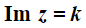on an Argand diagram?

• All complex numbers,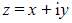, that satisfy the equation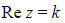lie on a vertical line with Cartesian equation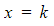• Any complex number along this vertical line will have a real part of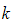• All complex numbers,, that satisfy the equation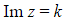lie on a horizontal line with Cartesian equation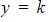• Any complex number along this horizontal line will have an imaginary part of• E.g. The lociand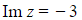are represented by the vertical line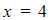and the horizontal line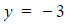Sketching the loci ofand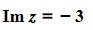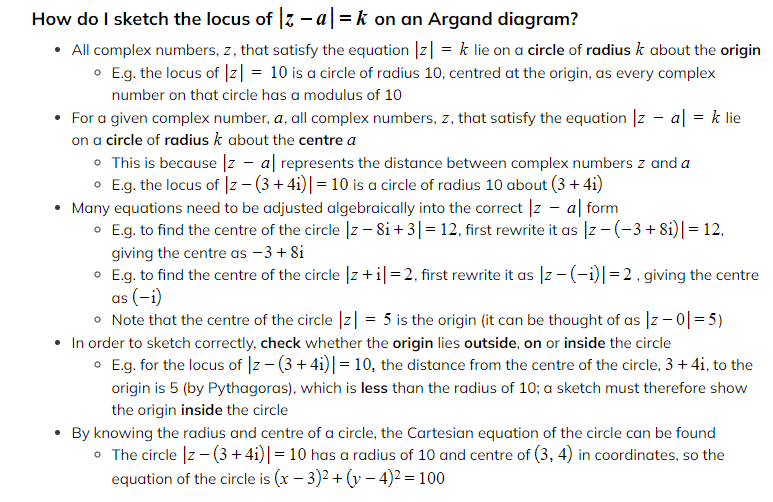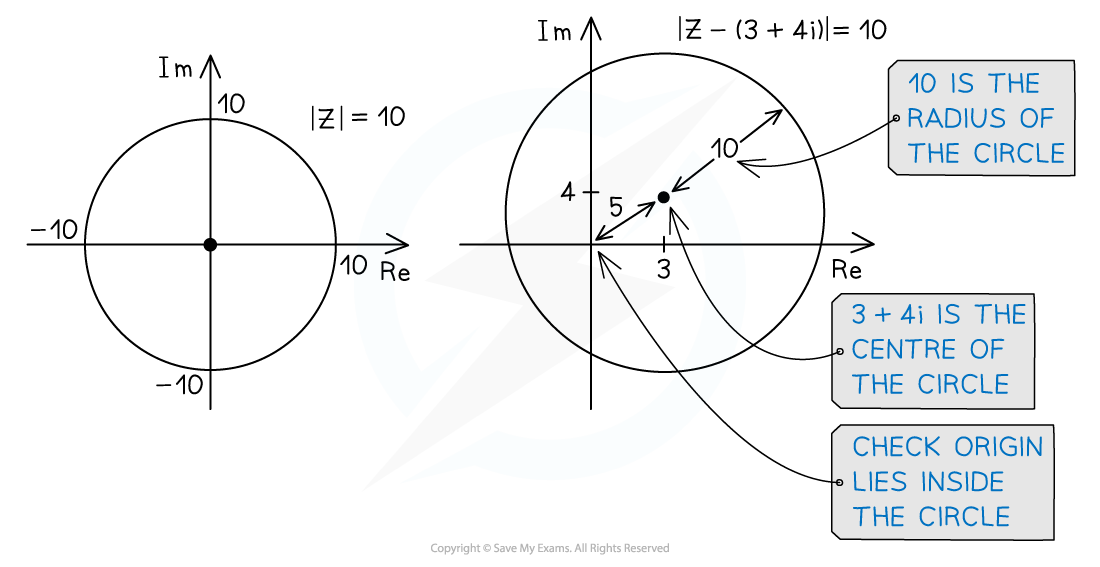####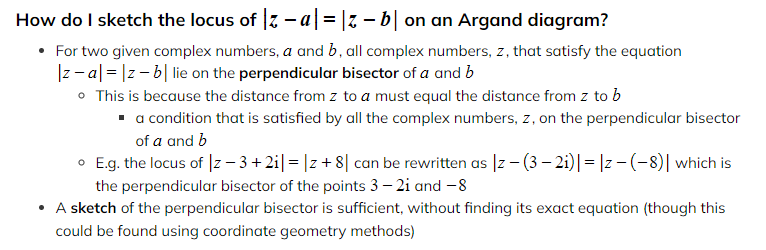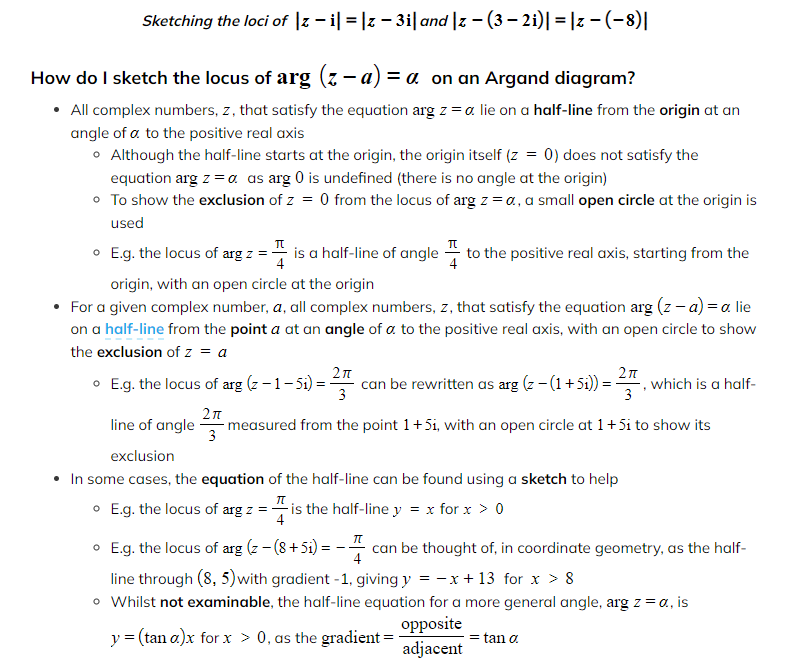#### Worked Example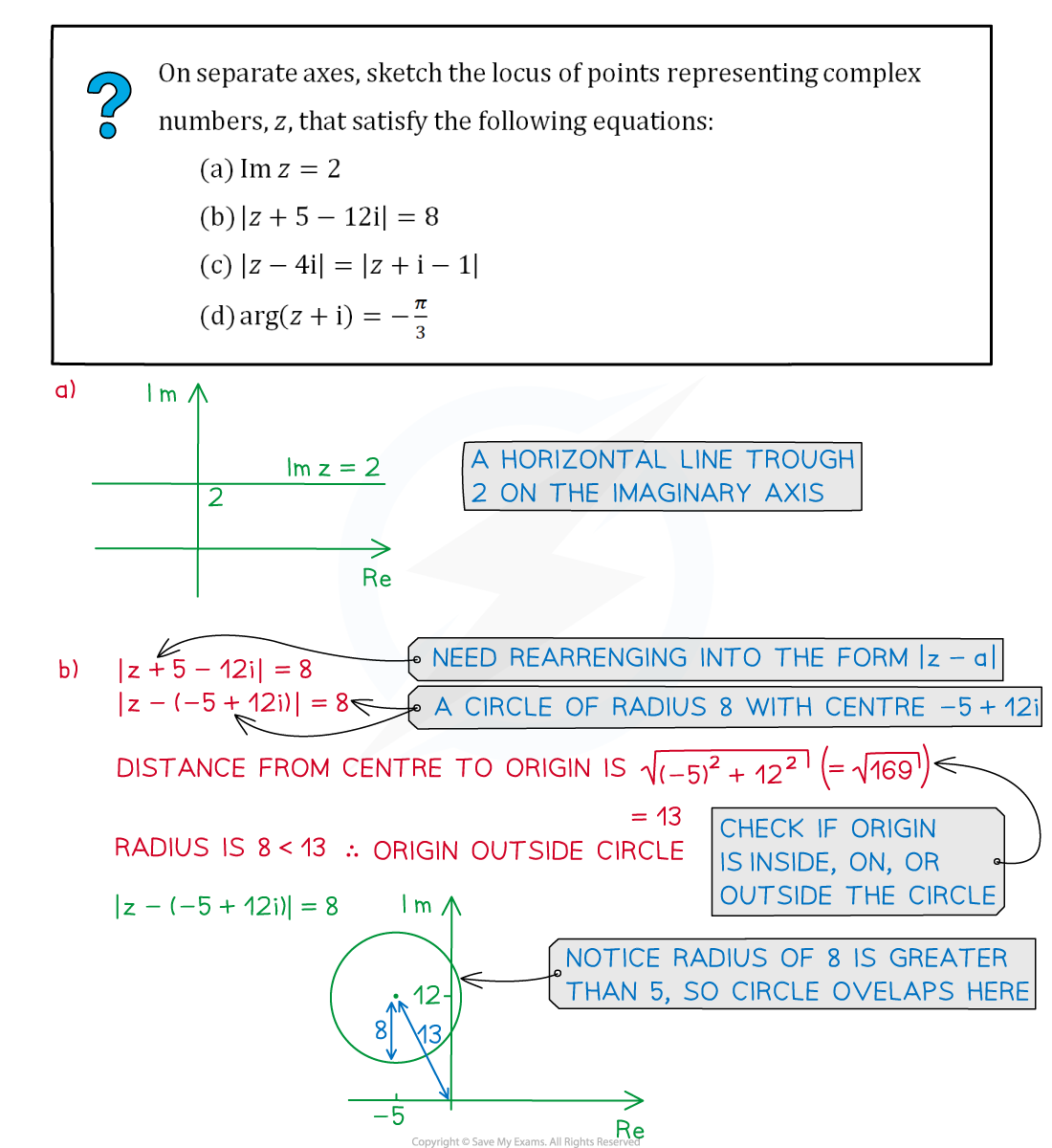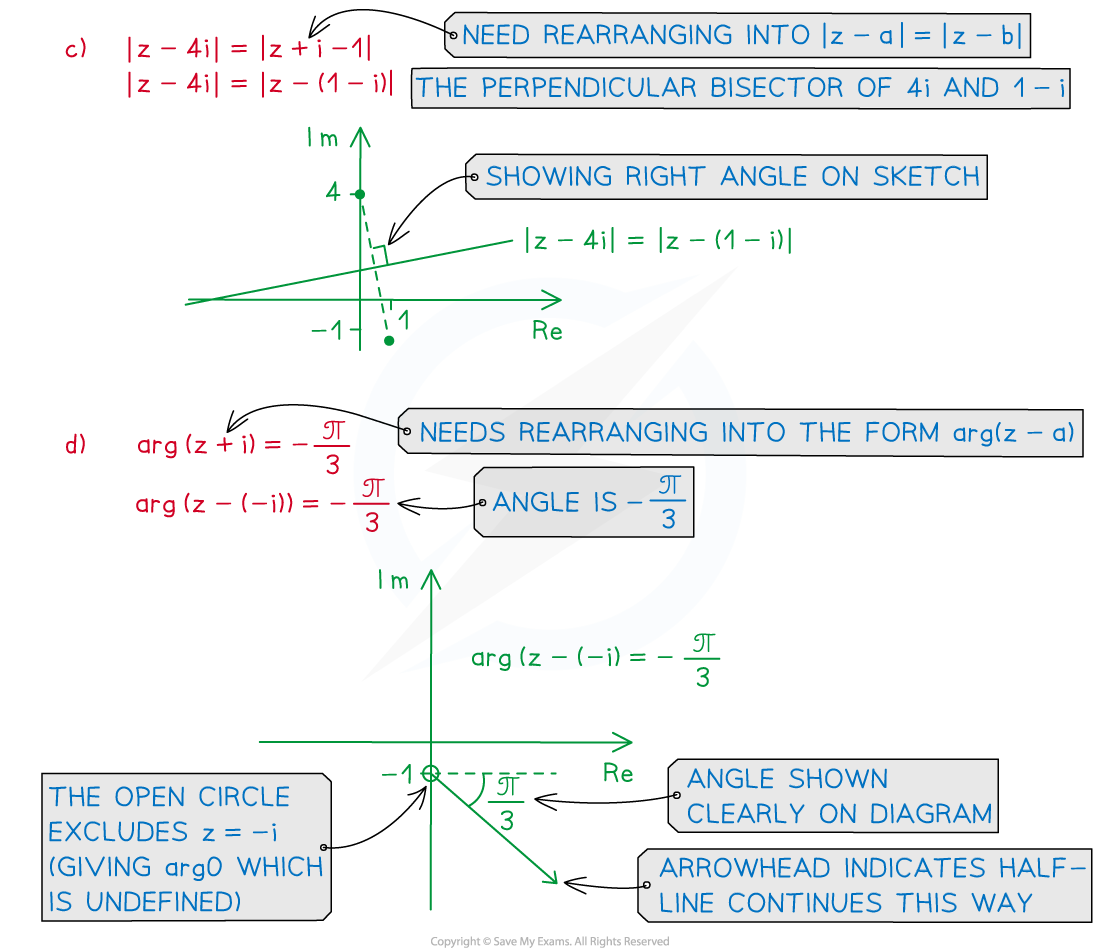#### Exam Tip

• In the exam, do not worry about making your diagrams perfect.
• A quick sketch with all the key features is sufficient.